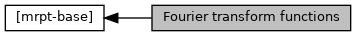Main MRPT website > C++ reference for MRPT 1.5.7
Fourier transform functions

## Detailed Description

Collaboration diagram for Fourier transform functions:## Functions

void BASE_IMPEXP mrpt::math::fft_real (CVectorFloat &in_realData, CVectorFloat &out_FFT_Re, CVectorFloat &out_FFT_Im, CVectorFloat &out_FFT_Mag)
Computes the FFT of a 2^N-size vector of real numbers, and returns the Re+Im+Magnitude parts. More...

void BASE_IMPEXP mrpt::math::dft2_real (const CMatrixFloat &in_data, CMatrixFloat &out_real, CMatrixFloat &out_imag)
Compute the 2D Discrete Fourier Transform (DFT) of a real matrix, returning the real and imaginary parts separately. More...

void BASE_IMPEXP mrpt::math::idft2_real (const CMatrixFloat &in_real, const CMatrixFloat &in_imag, CMatrixFloat &out_data)
Compute the 2D inverse Discrete Fourier Transform (DFT) More...

void BASE_IMPEXP mrpt::math::dft2_complex (const CMatrixFloat &in_real, const CMatrixFloat &in_imag, CMatrixFloat &out_real, CMatrixFloat &out_imag)
Compute the 2D Discrete Fourier Transform (DFT) of a complex matrix, returning the real and imaginary parts separately. More...

void BASE_IMPEXP mrpt::math::idft2_complex (const CMatrixFloat &in_real, const CMatrixFloat &in_imag, CMatrixFloat &out_real, CMatrixFloat &out_imag)
Compute the 2D inverse Discrete Fourier Transform (DFT). More...

void BASE_IMPEXP mrpt::math::cross_correlation_FFT (const CMatrixFloat &A, const CMatrixFloat &B, CMatrixFloat &out_corr)
Correlation of two matrixes using 2D FFT. More...

## ◆ cross_correlation_FFT()

 void mrpt::mrpt::math::cross_correlation_FFT ( const CMatrixFloat & A, const CMatrixFloat & B, CMatrixFloat & out_corr )

Correlation of two matrixes using 2D FFT.

Definition at line 1991 of file math.cpp.

## ◆ dft2_complex()

 void mrpt::math::dft2_complex ( const CMatrixFloat & in_real, const CMatrixFloat & in_imag, CMatrixFloat & out_real, CMatrixFloat & out_imag )

Compute the 2D Discrete Fourier Transform (DFT) of a complex matrix, returning the real and imaginary parts separately.

Parameters
 in_real The N_1xN_2 matrix with the real part. in_imag The N_1xN_2 matrix with the imaginary part. out_real The N_1xN_2 output matrix which will store the real values (user has not to initialize the size of this matrix). out_imag The N_1xN_2 output matrix which will store the imaginary values (user has not to initialize the size of this matrix). If the dimensions of the matrix are powers of two, the fast fourier transform (FFT) is used instead of the general algorithm.
fft_real, idft2_complex,dft2_real

Definition at line 1451 of file math.cpp.

## ◆ dft2_real()

 void mrpt::math::dft2_real ( const CMatrixFloat & in_data, CMatrixFloat & out_real, CMatrixFloat & out_imag )

Compute the 2D Discrete Fourier Transform (DFT) of a real matrix, returning the real and imaginary parts separately.

Parameters
 in_data The N_1xN_2 matrix. out_real The N_1xN_2 output matrix which will store the real values (user has not to initialize the size of this matrix). out_imag The N_1xN_2 output matrix which will store the imaginary values (user has not to initialize the size of this matrix).
fft_real, ifft2_read, fft2_complex If the dimensions of the matrix are powers of two, the fast fourier transform (FFT) is used instead of the general algorithm.

Definition at line 1186 of file math.cpp.

References MRPT_END, MRPT_START, and mrpt::math::rdft2d().

## ◆ fft_real()

 void mrpt::math::fft_real ( CVectorFloat & in_realData, CVectorFloat & out_FFT_Re, CVectorFloat & out_FFT_Im, CVectorFloat & out_FFT_Mag )

Computes the FFT of a 2^N-size vector of real numbers, and returns the Re+Im+Magnitude parts.

fft2_real

Definition at line 1004 of file math.cpp.

## ◆ idft2_complex()

 void mrpt::math::idft2_complex ( const CMatrixFloat & in_real, const CMatrixFloat & in_imag, CMatrixFloat & out_real, CMatrixFloat & out_imag )

Compute the 2D inverse Discrete Fourier Transform (DFT).

Parameters
 in_real The N_1xN_2 input matrix with real values, where both dimensions MUST BE powers of 2. in_imag The N_1xN_2 input matrix with imaginary values, where both dimensions MUST BE powers of 2. out_real The N_1xN_2 output matrix for real part (user has not to initialize the size of this matrix). out_imag The N_1xN_2 output matrix for imaginary part (user has not to initialize the size of this matrix).
fft_real, dft2_real,dft2_complex If the dimensions of the matrix are powers of two, the fast fourier transform (FFT) is used instead of the general algorithm.

Definition at line 1560 of file math.cpp.

## ◆ idft2_real()

 void mrpt::math::idft2_real ( const CMatrixFloat & in_real, const CMatrixFloat & in_imag, CMatrixFloat & out_data )

Compute the 2D inverse Discrete Fourier Transform (DFT)

Parameters
 in_real The N_1xN_2 input matrix with real values. in_imag The N_1xN_2 input matrix with imaginary values. out_data The N_1xN_2 output matrix (user has not to initialize the size of this matrix). Note that the real and imaginary parts of the FFT will NOT be checked to assure that they represent the transformation of purely real data. If the dimensions of the matrix are powers of two, the fast fourier transform (FFT) is used instead of the general algorithm.
 Page generated by Doxygen 1.8.14 for MRPT 1.5.7 Git: 8277875f6 Mon Jun 11 02:47:32 2018 +0200 at lun oct 28 01:50:49 CET 2019